# 用Python访问IBM i

“ IBM i 上执行一个 Python 程序”一文介绍了如何在 IBM i 上安装配置 Python 运行环境，下面利用这个机会介绍如何用 Python 去访问 IBM i 。

1. 用 Python 执行 IBM i 的命令。

1. 用 Python 访问 Db2 for IBM i 数据库。

1. 用 Python 执行 IBM i 上的命令

1. 导入类（ Import the class ）
2. 建立到 IBM i 的连接（ connect ）
3. 设置要执行的 CL 命令（ Set the CL command)
4. 调用要执行的 CL 命令（ Call ）
5. 关闭连接（ close connection ）
``````**WRKSYSSTS**

#coding=utf-8

from itoolkit import *

from itoolkit.transport import DatabaseTransport

import ibm_db_dbi as dbi

doc=open('/test/wajout.txt','w')

conn = dbi.connect()

itransport = DatabaseTransport(conn)

itool = iToolKit()

itool.call(itransport)

wrksyssts = itool.dict_out('wrksyssts')

print(wrksyssts['wrksyssts'])

print(wrksyssts['wrksyssts'],file=doc)

conn.close()
``````

，右侧的一部分没有显示出来。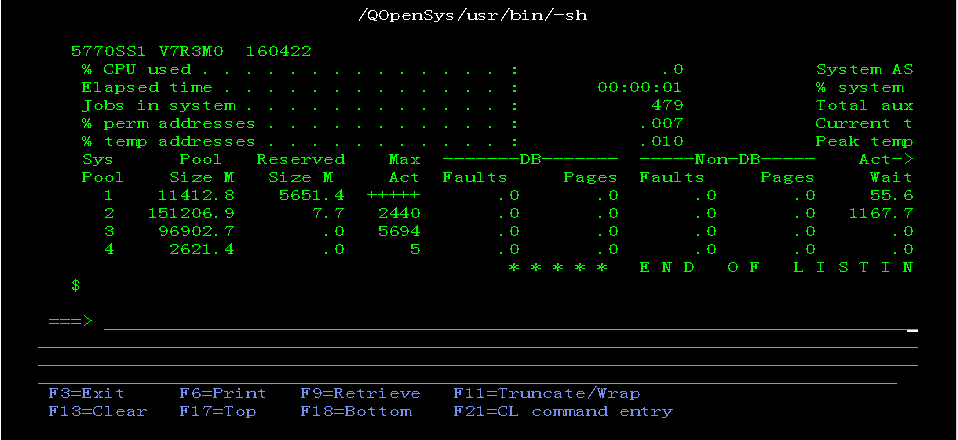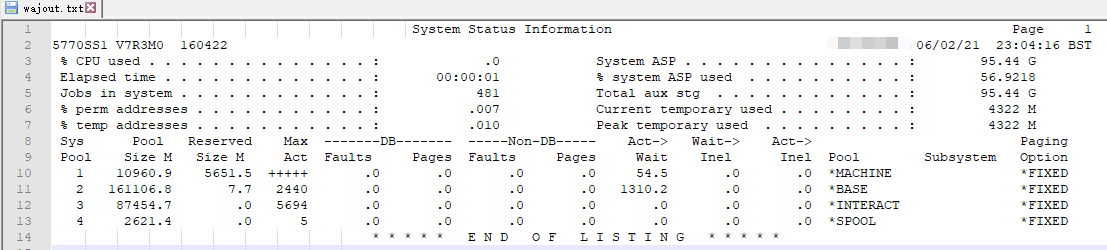1. 用 Python 访问 Db2 for IBM i 数据库。

1. 导入类（ Import the class ）
2. 连接数据库（ connect ）
3. 打开游标（ Open the cursor)
4. 设置要执行的 SQL 语句（ Set the cursor)
6. 关闭游标（ close cursor ）
7. 关闭数据库连接（ close connection ）

``````
#coding=utf-8

import ibm_db_dbi as dbi

conn = dbi.connect()

sql="select * from xqlib/tablib1"

con1 = conn.cursor()

con1.execute(sql)

print("\n\n ****** Start ******\n\n")

for row in con1.fetchall():

print(row)

con1.close()

conn.close()

print("\n\n ****** End ******\n\n")
``````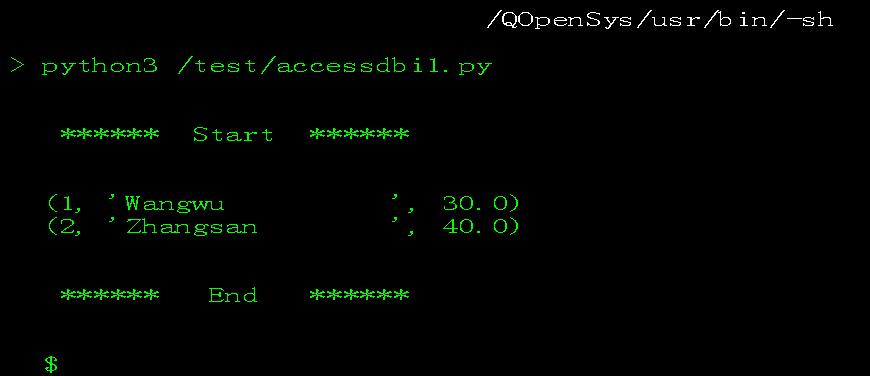``````#coding=utf-8

import ibm_db_dbi as dbi

from prettytable import from_db_cursor

conn = dbi.connect()

sql="select idn,name,age from xqlib.table1"

con1 = conn.cursor()

con1.execute(sql)

print(from_db_cursor(con1))

con1.close()

conn.close()``````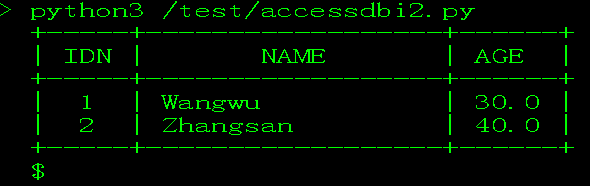``````#coding=utf-8

import ibm_db_dbi as dbi

from xlsxwriter import Workbook

conn = dbi.connect()

sql="select idn,name,age from xqlib.table1"

con1 = conn.cursor()

con1.execute(sql)

with Workbook('/test/table1.xlsx') as workbook:

worksheet.write_row('A1',['ID','Name','Age'])

for rownum,row in enumerate(con1,start=1):

worksheet.write_row(rownum,0,row)

con1.close()

conn.close()
``````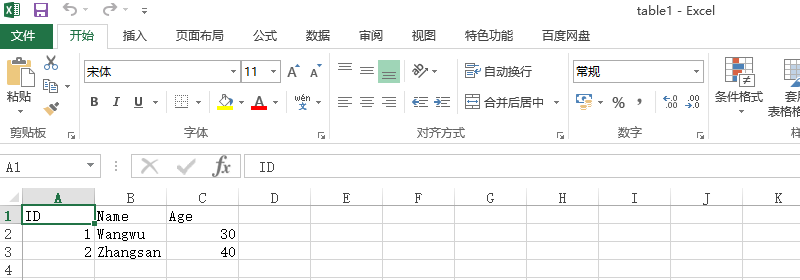``````
#coding=utf-8

import ibm_db_dbi as dbi

from xlsxwriter import Workbook

conn = dbi.connect()

sql="select name,age from xqlib.table1"

con1 = conn.cursor()

con1.execute(sql)

with Workbook('/test/table2.xlsx') as workbook:

worksheet.write_row('A1',['Name','Age'])

for rownum,row in enumerate(con1,start=1):

worksheet.write_row(rownum,0,row)

chart.set_title({'name':'Student Stastics'})

'name':  ' 年龄 ',

'categories':  '=Sheet1!\$A\$2:\$A'+ str(rownum+1),

'values':  '=Sheet1!\$B\$2:\$B'+ str(rownum+1)

})

worksheet.insert_chart('A5',chart)

con1.close()

conn.close()``````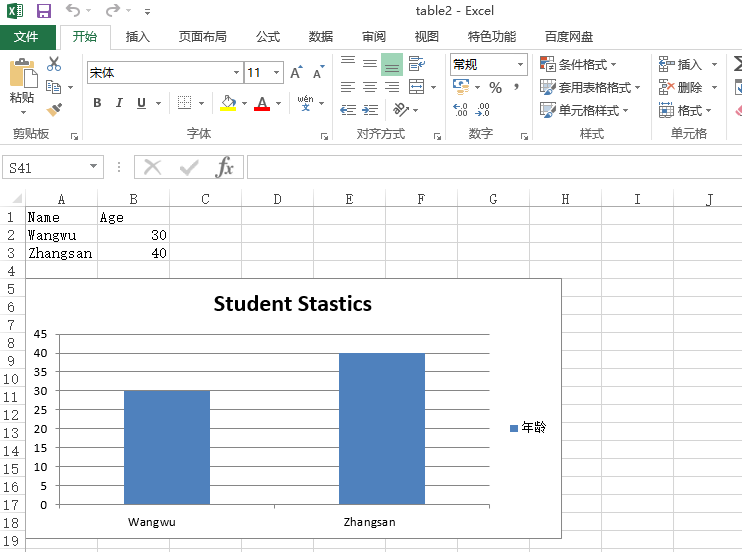“ IBM i 上执行一个 Python 程序”

Ctrl+Enter 发表